# Is Your Option Strategy Long Gamma Or Short Gamma?

You may know your option structure well enough to see whether it is short or long delta, theta, and vega.

But do you know if it is long gamma or short gamma?

And why does it matter?

Let’s find out.

The best way to introduce the topic of gamma is through examples.

## Contents

• Long Call Is Long Gamma
• Long Put Is Long Gamma
• The Short Put And Call is Short Gamma
• Gamma Of Stock
• Gamma Of Bull Put Spreads
• The Greeks
• Conclusion

## Long Call Is Long Gamma

A long call means an investor bought a call option. Its payoff diagram looks like this.

Date: Jan 10, 2022

Price: SPY at \$460

Buy one Feb 18 SPY \$460 call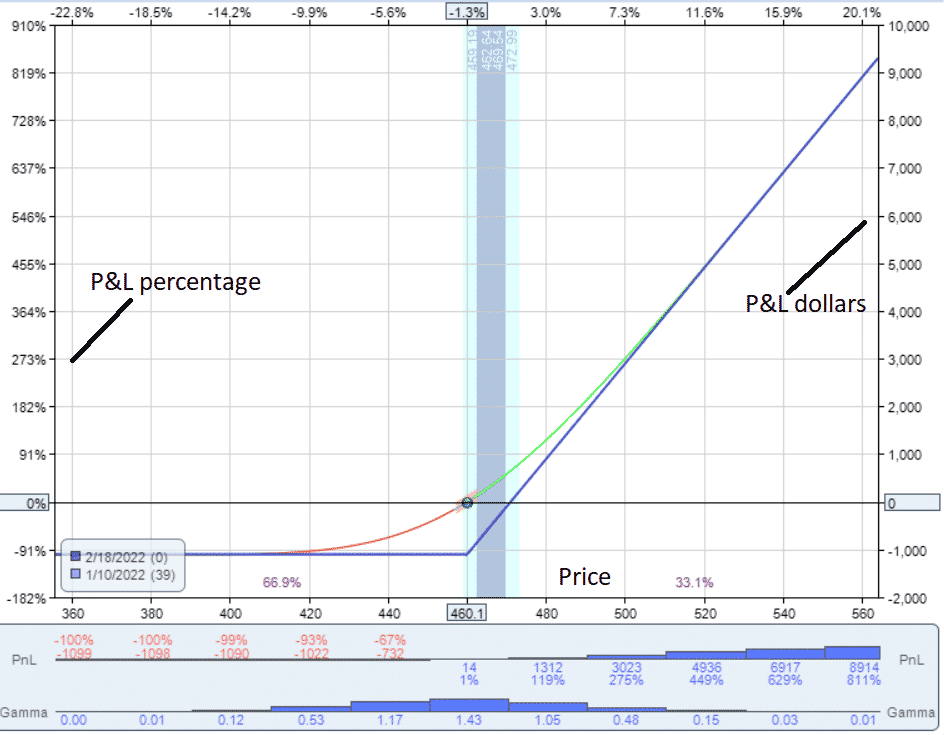It is a bullish strategy because we want the price to go up.

As the price goes up, the profit and loss (P&L) go up.

As noted in the histogram below the graph, the long call has positive gamma throughout its entire price range.

Right now, right after the call option was purchased, the gamma value is 1.43 when the underlying price is \$460.

The red/green curved line is the graph of what the P&L would be right now if the price were to move.

This line is known as the T+0 line.

The blue line is the P&L at expiration.

Right now, the price dot is at \$460.

If the price were to go up 20 points to \$480 right now, then the P&L would be \$1300.

If the price were to go down by 20 points to \$440, then according to the T+0 line, the P&L would be –\$700.

Let’s assume that there is a 50% chance that price might go 20 points up to \$480 and a 50% chance that price might go down by the same amount to \$440.

Then there is a 50% chance of winning \$1300 and a 50% chance of losing \$700.

This is the nature of having positive gamma.

Long gamma is a favorable characteristic to have.

## Long Put Is Long Gamma

Likewise, a long put option is also long gamma.

Date: Jan 10, 2022

Price: SPY at \$460

Buy one Feb 18 SPY put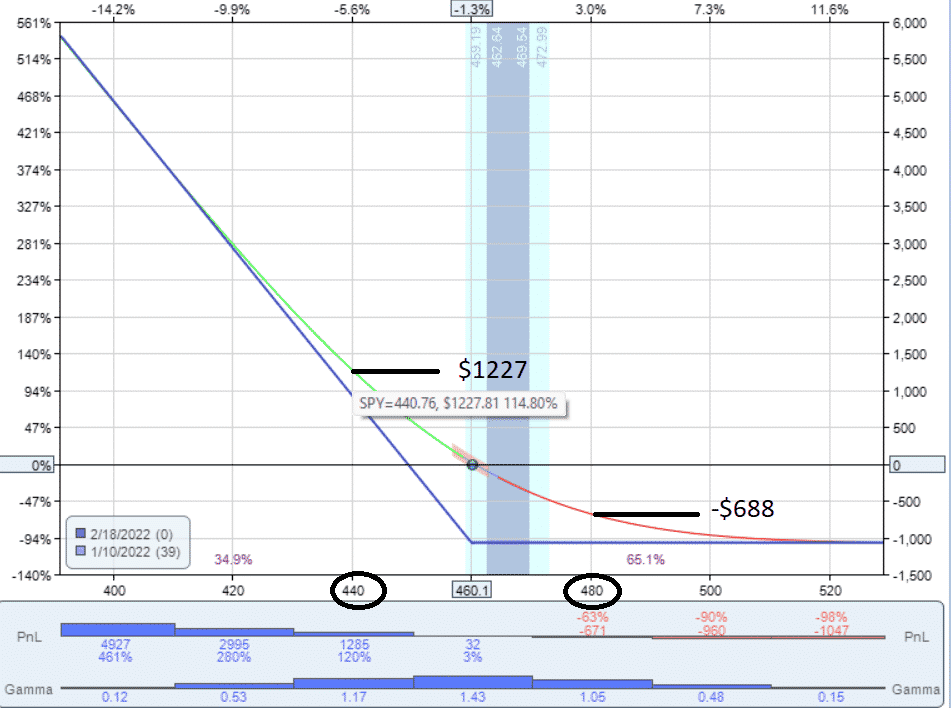This put option has a gamma of 1.43.

It is not a coincidence that this is the same value as the call option of the same strike and expiration.

But we won’t get into the topic of put-call parity now.

The long put is a bearish strategy, and we want the underlying price to go down.

Again, if the price makes a move down, the gain in profits will be greater than our loss if the price makes an equidistance move up.

This all has to do with the concavity of the T+0 curve.

The curvature is such because the expiration graph is capping the losses while not capping the gains.

## The Short Put And Call is Short Gamma

A short call means an investor sold a put option.

Date: Jan 10, 2022

Price: SPY at \$460

Sell one Feb 18 SPY \$460 put

This trade is a bullish strategy, as we want the underlying price to go up to the right of the graph.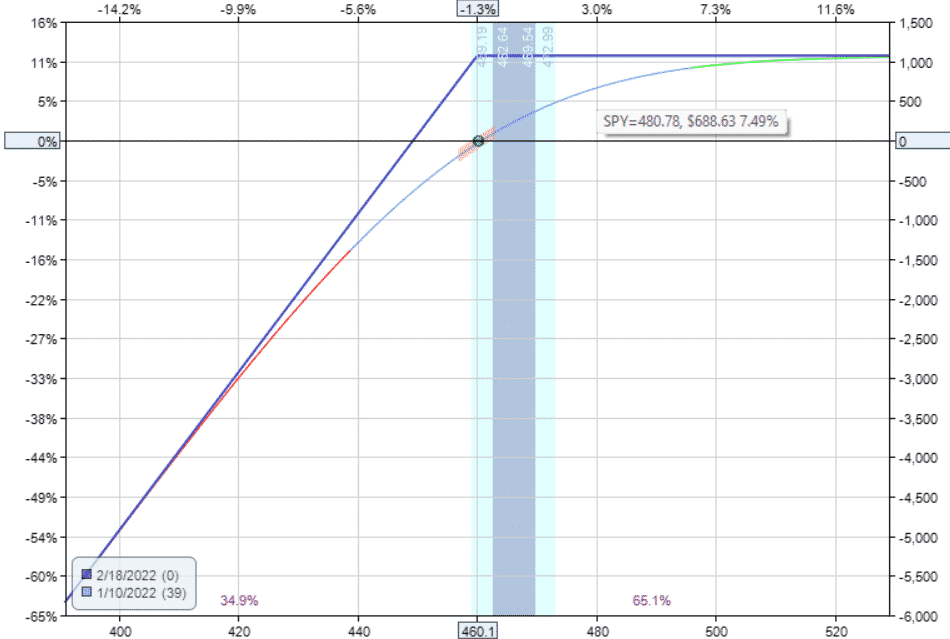The graph looks different, and the curvature of the T+0 line is different.

In this case, the gains are capped, but the losses are not.

As you lose money, you will lose more money faster.

It will be more difficult to make more money as you make money.

This is negative gamma at work.

We don’t like negative gamma.

The gamma is negative throughout its entire price range.

With the underlying price at \$460, the gamma value of the short put option is currently at -1.43.

It has the same magnitude but of opposite sign to the long put option of the same strike and expiration.

Similarly, the short call is short gamma — in other words, negative gamma.

## Gamma Of Stock

One hundred shares of stock have a delta of 1.0.

But it will not have any theta, vega, or gamma.

Therefore, 100 shares of SPY plus a long put will just have the gamma of the long put.

This “married put” position will be long gamma or have positive gamma.

Similarly, 100 shares of SPY plus a short call will have negative gamma.

This “covered call” position is short gamma.

## Gamma Of Bull Put Spreads

Suppose we have a bull put spread located around the 20-delta.

It consists of a short put and a long put option.

Date: Jan 10, 2022

Price: SPY at \$460

Sell one Feb 18 SPY \$435 put

Buy one Feb 18 SPY \$430 put

The gamma of the spread is the sum of the gamma of the two options.

And if you have multiple contracts, you multiple by that number of contracts.

Reading off the option, we see the \$430 put has a gamma value of 1.14.

The \$435 put has a gamma value of 1.23.

But since we are selling this option, we reverse the sign.

The short \$435 put has a gamma of -1.23.

In this case, the spread has a gamma of -0.09.

You can get this value looking at your trading platform or just taking the sum of 1.14 and -1.23.

The bull put spread is short gamma, but its magnitude is quite small.

The gamma of each option leg almost cancels each other out.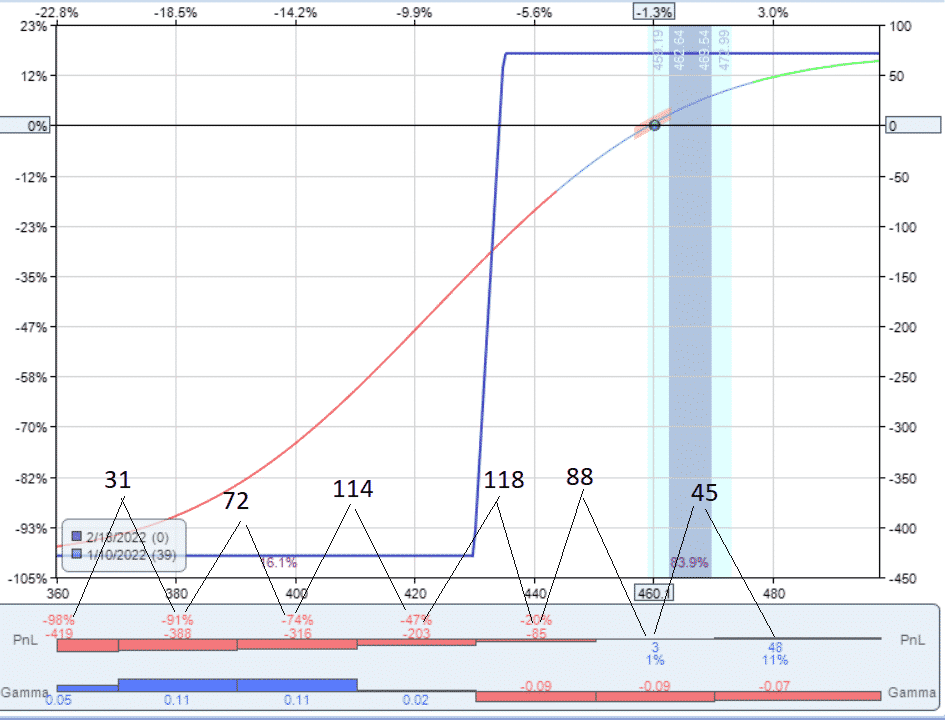The PnL histogram shows how P&L (profit and loss) changes as price changes.

If the price goes down from \$480 to \$460, the profit will drop from \$48 to \$3, which is s is a reduction in 45 profit dollars.

I’ve calculated and annotated the difference in the P&L for each 20 point change in price.

Note that the loss in P&L accelerates and gets bigger as the price goes from \$480 to \$460 and then from \$460 to \$440.

Then from \$440 down to \$420, there is the biggest drop in P&L — a drop of \$118 in profits.

It is the snowball effect.

As the price dot rolls down from the top of the T+0 hill, it rolls faster and faster.

You can see this in the curvature of the T+0 line.

The slope gets steeper.

The steeper the slope, the faster money is lost.

## The Greeks

Delta is related to the slope of the T+0 line. A flat T+0 line that is neither rising nor falling has zero delta.

A positive delta is represented by a T+0 line that slopes from lower left to upper right of the payoff diagram.

A steeper T+0 line means a larger delta.

Gamma is the rate of change of delta.

A larger magnitude of gamma means that the slope of the T+0 line will change a lot even with a small price change.

The line will be curvier.

One characteristic of out-of-the-money credit spreads that you are seeing in this example is that, once the position starts losing money, the delta changes in such a way that the position loss more money faster.

The delta gets larger, and the slope of the T+0 line increases.

Also, once the position starts to make money from its initial starting point, it will then start to make less and less money. It starts getting tired, like pushing a boulder up a hill.

That very last dollar available in the credit spread is very hard to get (I almost have to wait till expiration).

The easy money is already taken.

This is the unfavorable characteristic of having negative gamma.

Knowing that credit spreads have negative gamma is why many traders take their profits when 50% of the maximum profit of the credit spread is achieved.

They are not going to wait around for that last half partly for the reasons of this negative gamma behavior.

Knowing that a position has negative gamma means that we have to do something fast when the position starts taking losses.

Otherwise, the losses will grow faster and faster. We don’t want the price dot to roll down the hill.

But if for some reason, price did roll all the way down the hill…

As the price drops below the spread, gamma changes from negative to positive (see above gamma histogram).

After the price has gone down past the credit spread, when the position is at a large loss, the gamma becomes positive.

At this point, the incremental amount of P&L loss per change in price as price goes against us is getting smaller and smaller, as noted in my annotations.

Because iron condors are just two credit spreads, they too will be negative gamma.

Now you know how to read the graph and histogram of the bull call debit spread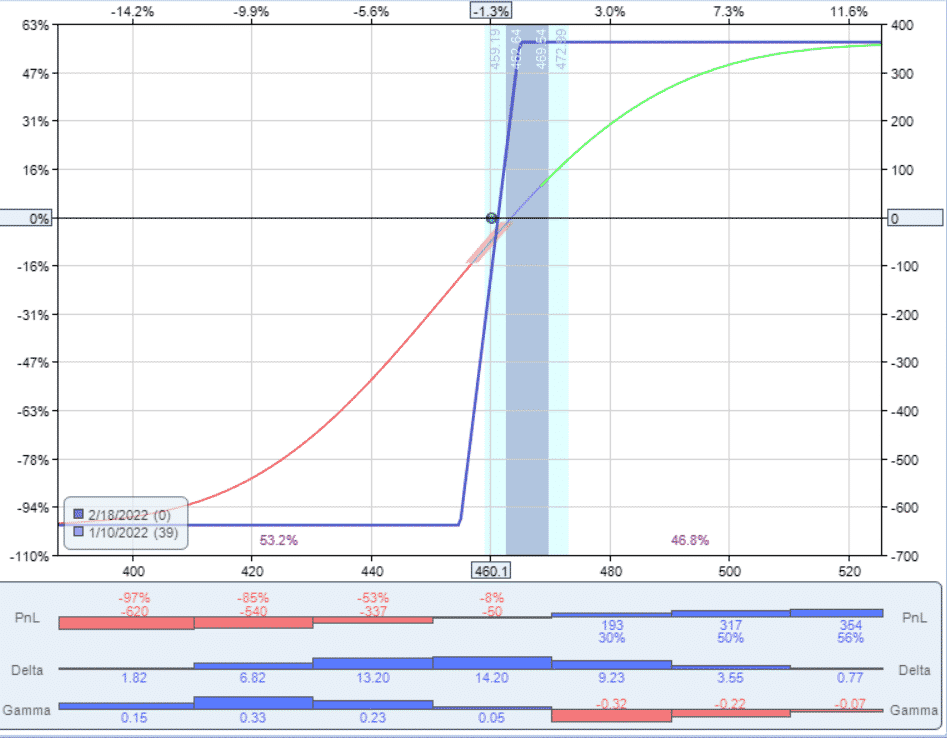As the position makes money (price dot moves to the right), it will make less and less money due to negative gamma.

As the price goes against the position, the loss will become less and less due to the effect of positive gamma.

## Conclusion

The long call and the long put have positive gamma throughout their entire price range.

This is a nice characteristic to have.

Does that mean that long calls and long puts are superior strategies?

Not really.

Each type of option has multiple characteristics such as theta and vega and others to consider.

The downside of the long calls and puts is that they have negative theta and lose money as time passes.

The more gamma you have, the more negative the theta becomes.

You get gamma at the expense of theta.

Many investors prefer to have positive theta over positive gamma.

The primary Greeks driving option prices are Delta, Vega, and Theta.

Gamma is a second-order Greek.

Is it possible to have positive theta and gamma at the same time?

In theory, no.

On rare occasions when the volatility skew curve is just right, it might exist in reality for a short time.

However, good luck finding them.